Mechanics

# Strength weight

Weight force is the gravitational force that any body that has mass exerts on another body that also has mass. It is an attractive force whose unit of measure is the newton and can be calculated from the product of the mass of the bodies that attract each other and the constant of universal gravitation, divided by the square of the distance that separates them.

## What is weight strength?

The weight force, also called gravitational force, is the attractive force between two or more bodies with mass . Although all bodies that have mass attract each other, the effect of the weight force is only noticeable in the vicinity of massive bodies such as planets and stars .

The weight force acting on us points towards the center of the Earth and is proportional to the magnitude of the local acceleration due to gravity .

## weight and mass

It is common that the words weight and mass are used indiscriminately, however it is important to note that the weight of an object is the measure of the force with which it is attracted by a gravitational field, while its mass is the measure of its inertia or, still, , the amount of matter contained in it. In this way, the weight of an object on the Moon is different from its weight on Earth , even though its mass is the same in these two regions.

## weight strength formula

The weight force can be calculated through the product of the mass of the body that suffers the action of the weight force and the gravity value .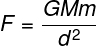P – weight force (N)

m – mass (kg)

g – acceleration due to gravity (m/s²)

In addition to the previous formula, the weight force can also be calculated using the law of universal gravitation. Watch:G – constant of universal gravitation (6.67.10-11 Nm²/kg²)

M in – masses of bodies (kg)

d – distance between bodies (m)

## weight force work

The work of the weight force is the calculation of the amount of energy that a body “loses” or “gains” when moving between two points of a gravitational field. It is important to emphasize that work only occurs when the displacement of the body is in the same direction as the weight force, that is, in the vertical direction, both in the positive direction, when the body acquires gravitational potential energy, and in the negative direction, when the body loses gravitational potential energy .

The work of the weight force can be calculated using the following expression: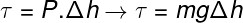τ – work (J)

P – weight force (N)

Δh – height variation (m)

From the formula represented above, it is possible to calculate the energy needed to lift a body of a certain mass to a desired height. Suppose we want to calculate how much energy is needed for a loaded elevator, whose total mass is 800 kg, to rise to a height of 30 m in a region where the acceleration due to gravity is 9.8 m/s².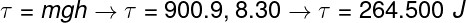Through the calculation, we estimate the amount of energy needed to raise a 900 kg elevator to a height of 30 m. This amount of energy is also known as gravitational potential energy.

## Normal strength and weight strength

The weight force and the normal force are forces of different origins that act on the same body . While the weight force originates from the gravitational attraction that the Earth makes on its surroundings, the normal force arises through the compression of a certain surface.

When we are sitting on the ground, for example, the force of weight pulls us “down” towards the Earth’s center of mass. The normal force, in turn, exerts an upward force on us, in the opposite direction to the force we exert against the ground. From this, it is possible to notice that the two forces act on the same body and that, therefore, they cannot be considered as an action-reaction pair .

## Weight strength exercises

Question 1  On the Moon, where the gravitational acceleration has a value of 1.6 m/s², an astronaut has a weight of 96 N. On Earth, where gravity is approximately 10 m/s², that astronaut’s weight would be equal to:

a) 800 N.

b) 600 N.

c) 500 N.

d) 400 N.

Resolution:

To solve the exercise, we first calculate the astronaut’s mass and then calculate his weight on Earth. Note the calculation: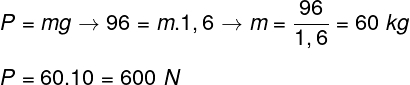Based on the calculation made above, the correct alternative is the letter B .

Question 2) Knowing that Earth’s gravity is worth, on average, 10 m/s², calculate what the weight of a body on Earth should be. Assume your weight on the Moon, where gravity is 1.6 m/s², is 160 N.

a) 1600 N

b) 1200 N

c) 1000 N

d) 800 N

Resolution:

To find out the body’s weight, we first find out its mass. Note the calculation: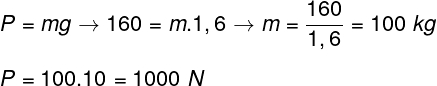Based on the resolution, the correct alternative is the letter C .